### D.46 De­riva­tion of the WKB ap­prox­i­ma­tion

The pur­pose in this note is to de­rive an ap­prox­i­mate so­lu­tion to the Hamil­ton­ian eigen­value prob­lem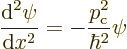where the clas­si­cal mo­men­tum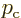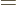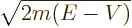is a known func­tion for given en­ergy. The ap­prox­i­ma­tion is to be valid when the val­ues of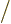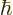are large. In quan­tum terms, you can think of that as due to an en­ergy that is macro­scop­i­cally large. But to do the math­e­mat­ics, it is eas­ier to take a macro­scopic point of view; in macro­scopic terms,is large be­cause Planck’s con­stantis so small.

Since ei­ther wayis a large quan­tity, for the left hand side of the Hamil­ton­ian eigen­value prob­lem above to bal­ance the right hand side, the wave func­tion must vary rapidly with po­si­tion. Some­thing that varies rapidly and non­triv­ially with po­si­tion tends to be hard to an­a­lyze, so it turns out to be a good idea to write the wave func­tion as an ex­po­nen­tial,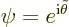and then ap­prox­i­mate the ar­gu­ment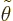of that ex­po­nen­tial.

To do so, first the equa­tion forwill be needed. Tak­ing de­riv­a­tives of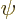us­ing the chain rule gives in terms of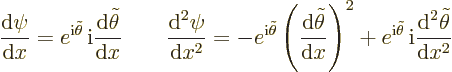Then plug­gingand its sec­ond de­riv­a­tive above into the Hamil­ton­ian eigen­value prob­lem and clean­ing up gives: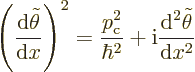(D.30)

For a given en­ergy,will de­pend on both what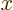is and whatis. Now, sinceis small, math­e­mat­i­cally it sim­pli­fies things if you ex­pandin a power se­ries with re­spect to: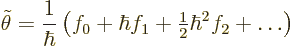You can think of this as writ­ing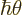as a Tay­lor se­ries in. The co­ef­fi­cients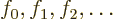will de­pend on. Sinceis small, the con­tri­bu­tion of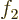and fur­ther terms tois small and can be ig­nored; only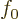and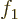will need to be fig­ured out.

Plug­ging the power se­ries into the equa­tion forpro­duces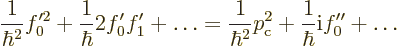where primes de­note-​de­riv­a­tives and the dots stand for pow­ers ofgreater than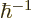that will not be needed. Now for two power se­ries to be equal, the co­ef­fi­cients of each in­di­vid­ual power must be equal. In par­tic­u­lar, the co­ef­fi­cients of 1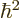must be equal,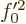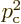, so there are two pos­si­ble so­lu­tions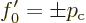For the co­ef­fi­cients of 1to be equal,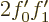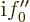, or plug­ging in the so­lu­tion for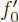,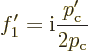It fol­lows that the-​de­riv­a­tive ofis given by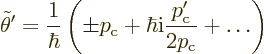and in­te­grat­ing givesas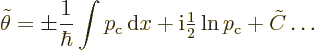where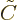is an in­te­gra­tion con­stant. Fi­nally,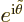now gives the two terms in the WKB so­lu­tion, one for each pos­si­ble sign, with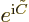equal to the con­stant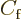or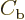.